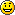categories:
archivies

September 22nd, 2006 | categorizilation: all categories,Kyrgyzstan

Distance / 距離： 34.31km
Time / 時間： 3h 45m
Average speed / 平均速度： 9.1km/h
Distance to date / 今日までの積算距離： 2692.1km

English Summary: Stopped early at a good campsite at the foot of the zig zags. But more importantly, time for you mathematicians to put your thinking caps on. The question of the day is, can you work out the gradient of a road using the variables ‘velocity’ and ‘altitude gain’. Example: I am cycling at a speed of 5km/h, and I am gaining altitude at a speed of 8 metres per minute. What is the gradient of the road I am on? I thought it had something to do with the amount of fies buzzing around my head divided by pie….mmmmm, pie…Pingback by 1 4 d e g r e e s » Day 64 - In Osh — September 25, 2006 @ 10:09 pm | post a comment

[...] If you need something to mull over while I hoof it over ‘the roof of the world’, then take a look at this post and let me know if you know the answer. [...]

OK Rob,

Your actual speed was 5km/hr = 5/60×1000 = 83m/min (make this the hypotenuse of the triangle).

The vertical side of the triangle is your rate of vertical ascent: 8m/min.

Using pythagorus, the bottom side of the triangle is:

x = sqrt(83^2-8^2) = 82.6m/min

Therefore, the gradient you were climbing is:

82.6 : 8

or

10.33 : 1

The angle of ascent was:

a = inverse-sin(8/83)

= 5.53 degrees.

Keep Him at the centre.Mike

try the boiling water thing the higher up you are the lower the boiling of water, so you need the following.

1x sat phone

2x stoves

2x kettles

2x thermomonometers

2x equal amounts of water from a constant source

2x fuel

when you've got all that sorted call me and I'll go to the seaside and do the experiment at the same time we can then compare notes and findings

also the world is not exactly square so I'm only guessing here but matey maths is wrong… right in principle but wrong in fact.

Mike, so what's that in percent? They have signs here that say 12% gradient for all the steep hills, but some feel steeper than others…

Carl, the world isn't square?! No wonder it was taking me so long to find an edge.

To find the gradient as a percentage you need to divide the vertical velocity by the horizontal velocity and then multiply by 100.

So for above:

8/82.6×100 = 9.69%

To get a more accurate result for the percentage gradient:

1) Zero your speedo at the bottom of the hill. Take note of the altitude at the bottom of the hill. Start your stop watch.

2) Bike up to the top of the hill.

3) At the top, stop your stop watch, take note of your average speed and the altitude you have climbed.

time = t (s)

average velocity = v (km/hr)

altitude climbed = a (m)

Plug the numbers into the following formula which I just derived:

———————–

———————–

(This is the simplified approximate version – which I recommend using, unless you like maths).

Alternatively use this nutty formula:

(This is the more precise version)

However note: this is an average gradient – if you want to know the gradient of the steepest part, you'll need to record "t", "v" & "a" over the steepest part.

This should give you something to think about on those crazy hills of yours!Mike

Professor Mike is a bit of a legend huh? But if you don't have a scientific calculator on you, you can plug some figures into here to make conversionshttp://www.cactus2000.de/uk/unit/massgrd.shtml

Unless you do the pythagoras equation, you will need to estimate your horizontal distance, but it shouldn't be too much less than the reading on your speedo.

blah balh blah still think it better with kettles of boiling water all this cheating with a watch what can tell you how high your getting whats that all about next you'll be telling you got a GPS?

whatever happend to lady luck and using the stars?

8m/min / (5kph/60*1000)= 8/83.33= 0.0960m/m

asin(0.0960)=5.5089 degree slope

tan(5.5089 deg) = .0964 m-vertical/m-horizontal